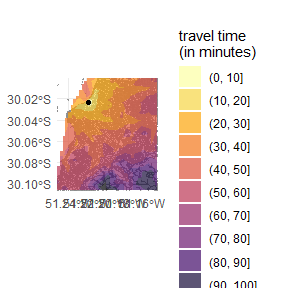# Calculating and visualizing Isochrones

#### 2023-01-27

Abstract

This vignette shows how to calculate and visualize isochrones in R using the r5r package.

# 1. Introduction

An isochrone of a given place includes all the areas reachable from that place within a certain amount of time. This vignette shows how to calculate and visualize isochrones in R using the r5r package.

In this reproducible example, we will be using a sample data set for the city of Porto Alegre (Brazil) included in r5r. Our aim here is to calculate several isochrones departing from the central bus station given different travel time thresholds. We’ll do this in 4 quick steps:

1. Increase Java memory and load libraries
2. Build routable transport network
3. Calculate travel times
4. Map Isochrones

# 2. Build routable transport network with setup_r5()

### Increase Java memory and load libraries

Before we start, we need to increase the memory available to Java and load the packages used in this vignette.

options(java.parameters = "-Xmx2G")

library(r5r)
library(sf)
library(data.table)
library(ggplot2)
library(interp)
library(dplyr)

To build a routable transport network with r5r and load it into memory, the user needs to call setup_r5 with the path to the directory where OpenStreetMap and GTFS data are stored.

# system.file returns the directory with example data inside the r5r package
# set data path to directory containing your own data if not using the examples
data_path <- system.file("extdata/poa", package = "r5r")

r5r_core <- setup_r5(data_path)

# 3. Calculate travel times

In this example, we will be calculating the travel times by public transport from the central bus station in Porto Alegre to every other block in the city. With the code below we compute multiple travel time estimates departing every minute over a 120-minute time window, between 2pm and 4pm.

# read all points in the city

# subset point with the geolocation of the central bus station
central_bus_stn <- points[291,]

# routing inputs
mode <- c("WALK", "TRANSIT")
max_walk_time <- 30 # in minutes
max_trip_duration <- 120 # in minutes
departure_datetime <- as.POSIXct("13-05-2019 14:00:00",
format = "%d-%m-%Y %H:%M:%S")

time_window <- 120 # in minutes
percentiles <- 50

# calculate travel time matrix
ttm <- travel_time_matrix(r5r_core,
origins = central_bus_stn,
destinations = points,
mode = mode,
departure_datetime = departure_datetime,
max_walk_time = max_walk_time,
max_trip_duration = max_trip_duration,
time_window = time_window,
percentiles = percentiles,
progress = FALSE)

#>            from_id           to_id travel_time_p50
#> 1: 89a90128a8fffff 89a901291abffff              61
#> 2: 89a90128a8fffff 89a9012a3cfffff              84
#> 3: 89a90128a8fffff 89a901295b7ffff              63
#> 4: 89a90128a8fffff 89a901284a3ffff              66
#> 5: 89a90128a8fffff 89a9012809bffff              55
#> 6: 89a90128a8fffff 89a901285cfffff              45

# 4. Map Isochrones

Now we only need to organize the travel time matrix output ttm and plot it on the map.

# extract OSM network
street_net <- street_network_to_sf(r5r_core)

# add coordinates of destinations to travel time matrix
ttm[points, on=c('to_id' ='id'), :=(lon = i.lon, lat = i.lat)]

# interpolate estimates to get spatially smooth result
travel_times.interp <- with(na.omit(ttm), interp(lon, lat, travel_time_p50)) %>%
with(cbind(travel_time=as.vector(z),  # Column-major order
x=rep(x, times=length(y)),
y=rep(y, each=length(x)))) %>%
as.data.frame() %>% na.omit()

# find isochrone's bounding box to crop the map below
bb_x <- c(min(travel_times.interp$x), max(travel_times.interp$x))
bb_y <- c(min(travel_times.interp$y), max(travel_times.interp$y))

# plot
ggplot(travel_times.interp) +
geom_sf(data = street_net\$edges, color = "gray55", size=0.01, alpha = 0.7) +
geom_contour_filled(aes(x=x, y=y, z=travel_time), alpha=.7) +
geom_point(aes(x=lon, y=lat, color='Central bus\nstation'),
data=central_bus_stn) +
scale_fill_viridis_d(direction = -1, option = 'B') +
scale_color_manual(values=c('Central bus\nstation'='black')) +
scale_x_continuous(expand=c(0,0)) +
scale_y_continuous(expand=c(0,0)) +
coord_sf(xlim = bb_x, ylim = bb_y) +
labs(fill = "travel time\n(in minutes)", color='') +
theme_minimal() +
theme(axis.title = element_blank())### Cleaning up after usage

r5r objects are still allocated to any amount of memory previously set after they are done with their calculations. In order to remove an existing r5r object and reallocate the memory it had been using, we use the stop_r5 function followed by a call to Java’s garbage collector, as follows:

r5r::stop_r5(r5r_core)
rJava::.jgc(R.gc = TRUE)

If you have any suggestions or want to report an error, please visit the package GitHub page.